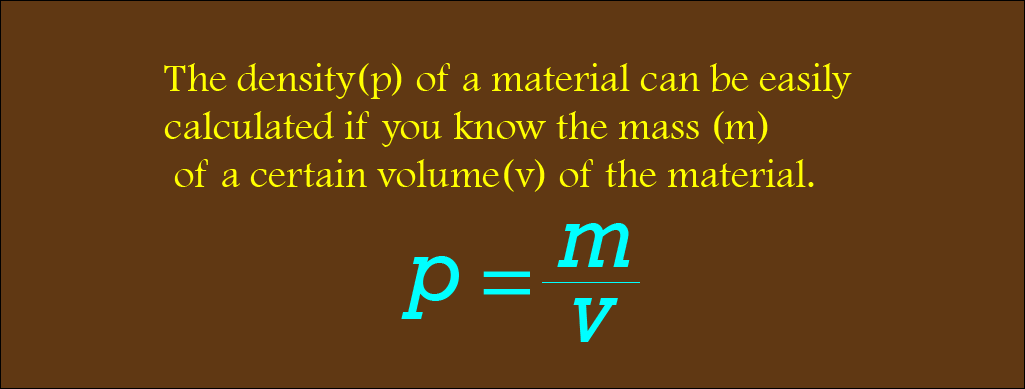# What is density? Density definition and Density formula

Published on 05-Sep-2022

## Density

Solids, liquids, and gases have different properties and characteristics. One such characteristic is density. Solids are often very dense; they have a high mass for a particular volume. Liquids are often less dense as they have a low mass for a specific volume, and gases have even lower densities.

## Density formula

The density(p) of a material can be easily calculated if you know the mass (m) of a certain volume(v) of the material.

### The equation is p = m/v.Before jumping into density, we need to know mass and volume briefly.

Mass: Mass is the amount of matter that a body contains by force exerted on it by the earth's gravitational field. Its unit is measured in kilograms (kg). Conversely, the force exerted by the earth's gravitational field is measured in a unit called newtons(N).

There is a way to find mass if the gravitational force(W) is given. We know that the earth's gravitational field strength(g) is 10 kg/N. W = mg. Weight = mass x 10.

Example: The weight of a carton of apple is 90 Newtons. What is its mass of it? Putting it into the formula, m = W/g, it comes m = 90/10 = 9kg.

Again, the weight of a carton of oranges is 80 Newtons. What is its mass of it? Putting it into the formula, m = W/g, it comes m = 80/10 = 8kg.

Again, the weight of a carton of mangoes is 70 Newtons. What is its mass of it? Putting it into the formula, m = W/g, it comes m = 70/10 = 7kg.

Volume: Volume is, in simple words, the amount of space taken by an object. It is measured in cubic units.

Now that we have entirely known about mass and volume, we are going to see some calculations relating to density.

Example: A piece of iron has a mass of 390kg and a volume of 0.05 m3. What is the density of the piece of iron? Putting it into the formula, = 390/0.05 = 7800 kg/m3.

A piece of wood has a mass of 400kg and a volume of 5 m3. What is the density of the piece of wood? Putting it into the formula, = 400/5 = 80 kg/m3.

A piece of glass has a mass of 380kg and a volume of 16 m3. What is the density of the piece of glass? Putting it into the formula, = 380/16 = 23.75 kg/m3.

Tag  #